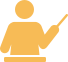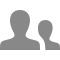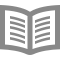## Mathematics for Machine Learning: PCA

Цена:49 USD

###### День проведения: По запросу

Тип: Электронное обучение

Категория: Анализ данных

Язык: Английский

Город: Online

### Описание тренинга:

This intermediate-level course introduces the mathematical foundations to derive Principal Component Analysis (PCA), a fundamental dimensionality reduction technique. We'll cover some basic statistics of data sets, such as mean values and variances, we'll compute distances and angles between vectors using inner products and derive orthogonal projections of data onto lower-dimensional subspaces. Using all these tools, we'll then derive PCA as a method that minimizes the average squared reconstruction error between data points and their reconstruction.

At the end of this course, you'll be familiar with important mathematical concepts and you can implement PCA all by yourself. If you?re struggling, you'll find a set of jupyter notebooks that will allow you to explore properties of the techniques and walk you through what you need to do to get on track. If you are already an expert, this course may refresh some of your knowledge. The lectures, examples and exercises require: 1. Some ability of abstract thinking 2. Good background in linear algebra (e.g., matrix and vector algebra, linear independence, basis) 3. Basic background in multivariate calculus (e.g., partial derivatives, basic optimization) 4. Basic knowledge in python programming and numpy Disclaimer: This course is substantially more abstract and requires more programming than the other two courses of the specialization. However, this type of abstract thinking, algebraic manipulation and programming is necessary if you want to understand and develop machine learning algorithms.
?

### WHAT YOU WILL LEARN

• Implement mathematical concepts using real-world data

• Derive PCA from a projection perspective

• Understand how orthogonal projections work

• Master PCA

### Дополнительная информация

SKILLS YOU WILL GAIN

• Dimensionality Reduction
• Python Programming
• Linear Algebra

### Выступающие:

Instructor rating3.84/5?(170 Ratings)### Marc Peter Deisenroth

Lecturer in Statistical Machine Learning

Department of Computing52,491?Learners1?Course

## Участие

Поделись с друзьями

## Информация об учебном центре

Тип компании: ОАО/ЗАО/ООО

Количество сотрудников: 500-1500